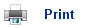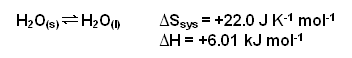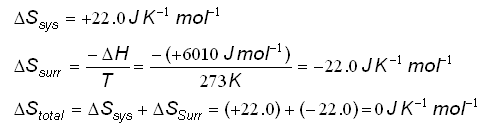# Entropy and Equilibrium

• According to the Second Law of Thermodynamics, a change takes place in the direction which corresponds to an increase inStotal.
• This is the case for the freezing of water below temperatures of 0oC; however, above this temperature theStotal for the freezing of ice is negative and so the reverse occurs; the ice melts.
• When theStotal is zero, there will be no tendency for the reaction to go in either direction; it will be at equilibrium.
• This can be shown once again for the freezing and melting of water:• At 273K, theStotal for the equilibrium reaction is:• The reaction is at equilibrium; liquid water and ice exist together at 0oC.

At equilibrium, theStotal is equal to zero.

• Even though theoretically the equilibrium occurs at 0oC, the temperature does not have to be exactly equal to this for it to occur.
• If the conditions happen to be standard, then the standard entropy change will be zero; however, the change rarely is zero at equilibrium.

## Useful books for revision

Revise A2 Chemistry for Salters (OCR A Level Chemistry B)Salters (OCR) Revise A2 ChemistryHome